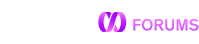# Condition with JSON - without knowledge of fields order

Hello, is possible to filter data by json without required order of parameters? For example I have table with json field and one row contains `{"a":1,"b":2}`

``````SELECT id FROM ab WHERE field = '{"a":1,"b":2}'; // ok
SELECT id FROM ab WHERE field = '{"b":2,"a":1}'; // not ok - but it is same json
``````

I found that mysql probably solve this by casting of json string as json … but how to solve this in memSQL? I cannot find anything in documentation. Thanks.

I know of 3 options:

``````MySQL [db]> create table t(a json);
MySQL [db]> insert into t values ('{"a":1, "b":1}'), ('{"b":1, "a":1}'), ('{"a":2, "b":2}'), ('{"b":2, "a":2}');
MySQL [db]> select * from t where a::a = 1 and a::b = 1;
+---------------+
| a             |
+---------------+
| {"a":1,"b":1} |
| {"a":1,"b":1} |
+---------------+

MySQL [db]> select * from t where a = '{"a":1,"b":1}';
+---------------+
| a             |
+---------------+
| {"a":1,"b":1} |
| {"a":1,"b":1} |
+---------------+

MySQL [db]> select * from t where a = '{"b":1,"a":1}':>json;
+---------------+
| a             |
+---------------+
| {"a":1,"b":1} |
| {"a":1,"b":1} |
+---------------+
``````

In order, they’re:

• Extract the fields you’re filtering on.
• Take advantage of the fact that JSON -> string conversion always lists keys in lexicographical order, and just ensure the string filter is likewise ordered.
• Cast the filter to JSON, as you mention.

I suspect that the first option will outperform the others for columnstore JSON, though that’s worth testing. Note that the semantics of the first option are different from the other two options, since the `a::a = .., b::b =` filter will succeed if the object contains more keys than just `a` and `b` while the other filters will fail if the object contains other keys:

``````MySQL [db]> select * from t where a = '{"b":1}':>json;
Empty set (0.001 sec)

MySQL [db]> select * from t where a::b = 1;
+---------------+
| a             |
+---------------+
| {"a":1,"b":1} |
| {"a":1,"b":1} |
+---------------+
2 rows in set (0.106 sec)``````

Perfect. Thanks … this `select * from t where a = '{"b":1,"a":1}':>json;` is exactly what I need.